Learn all Concepts of Polynomials Class 9 (with VIDEOS). Check - Polynomials Class 9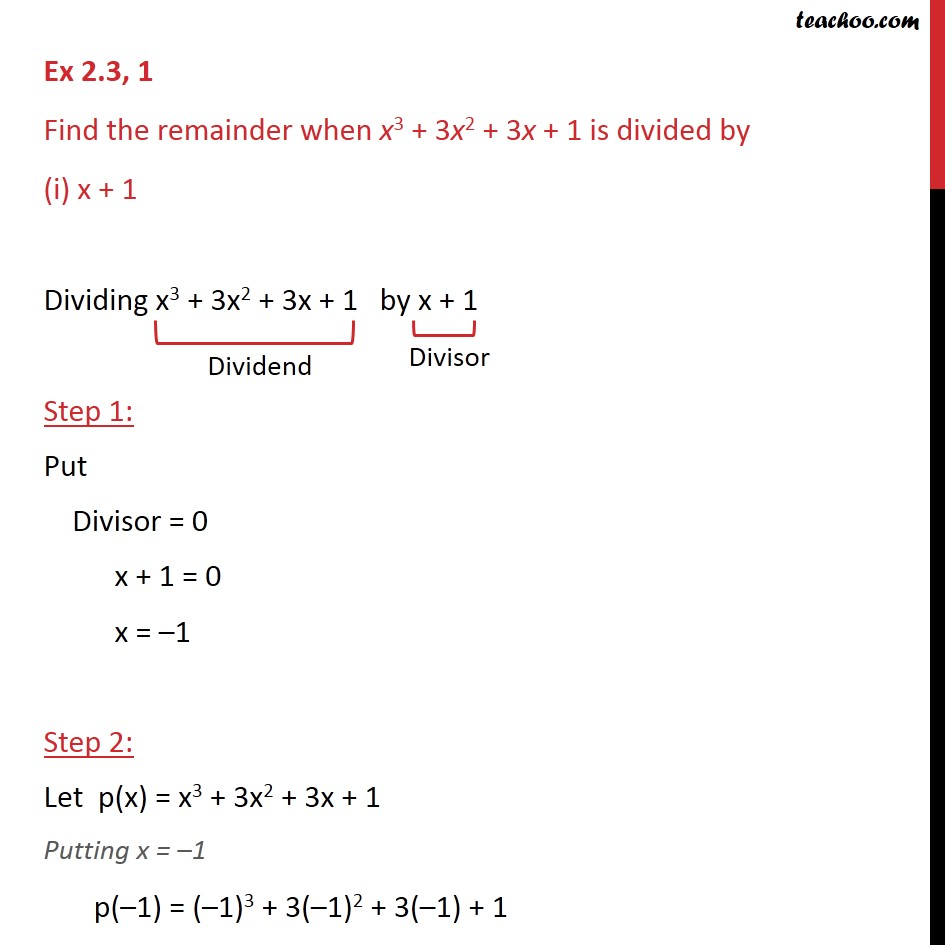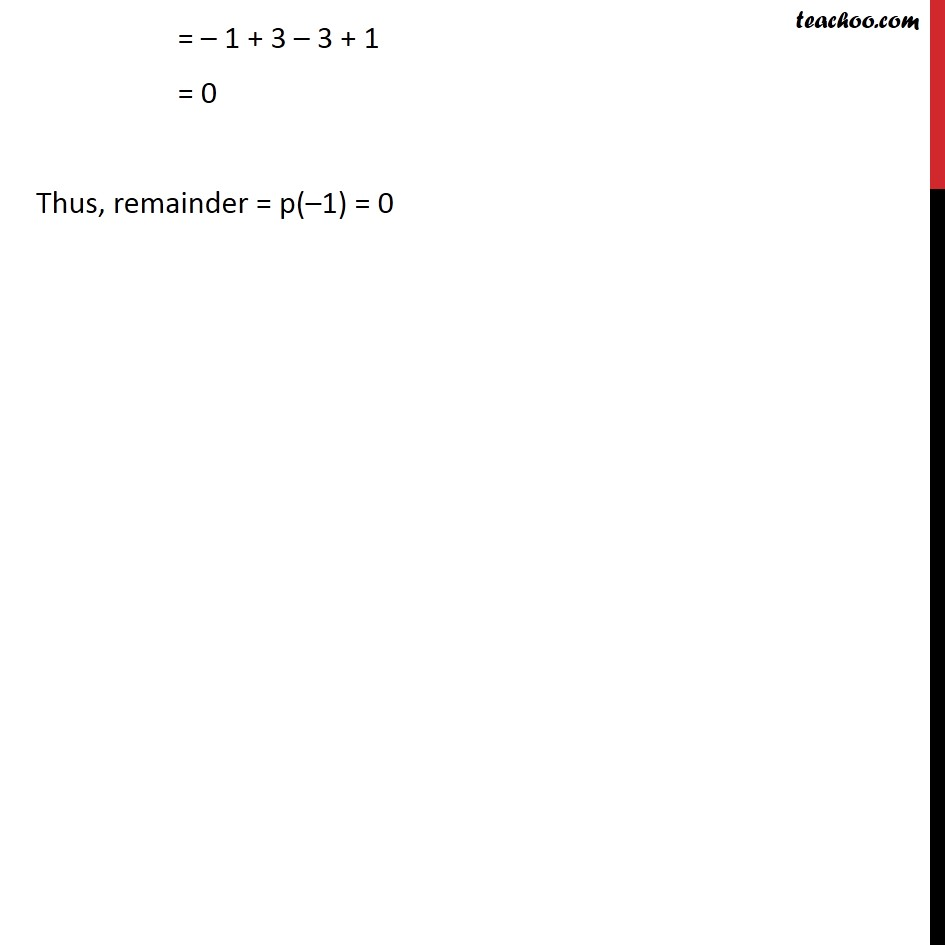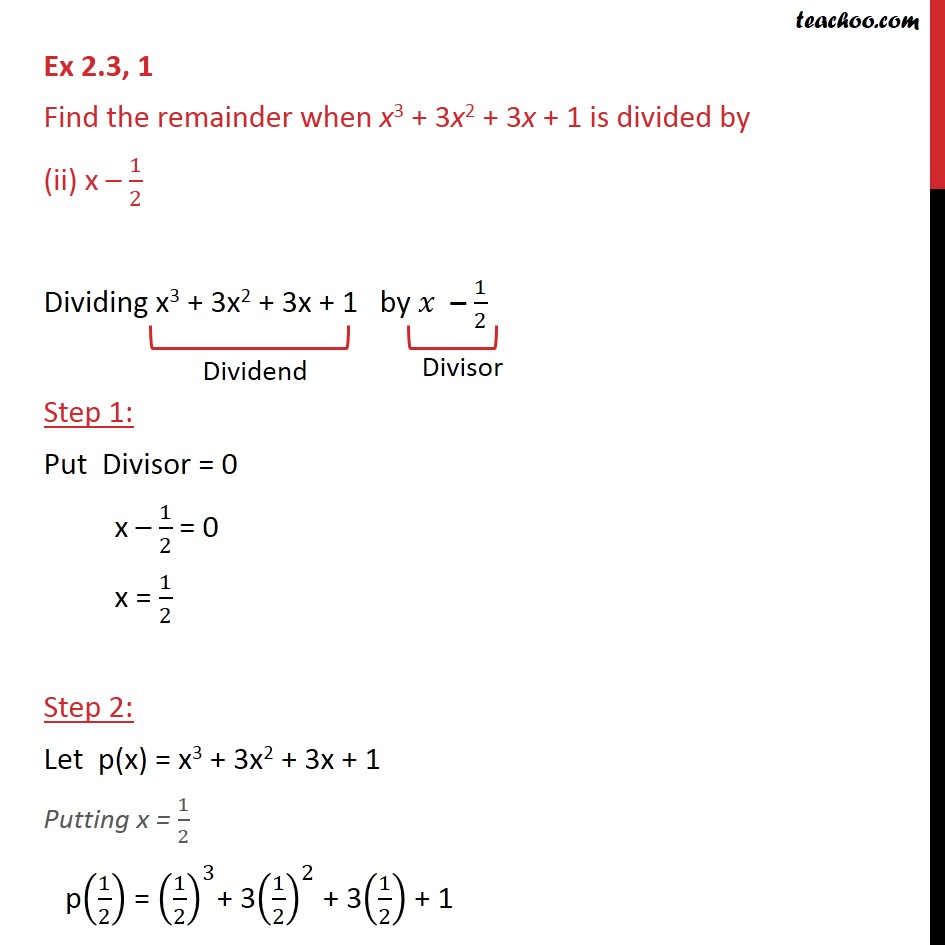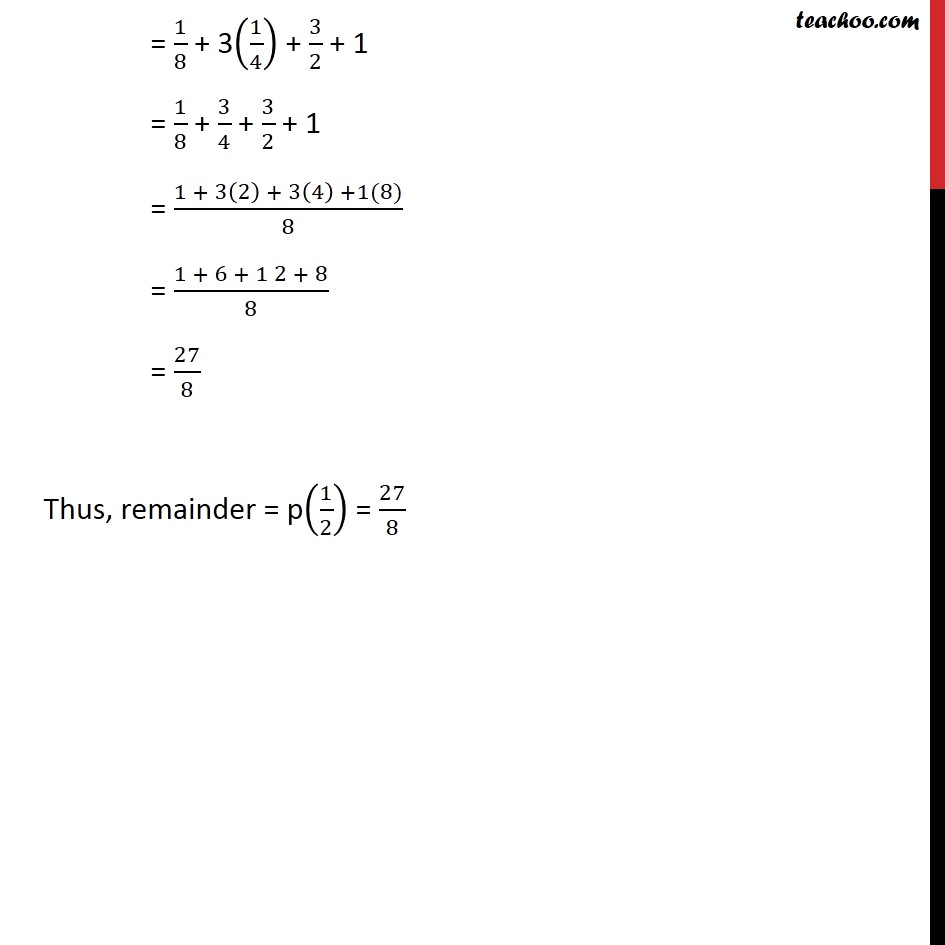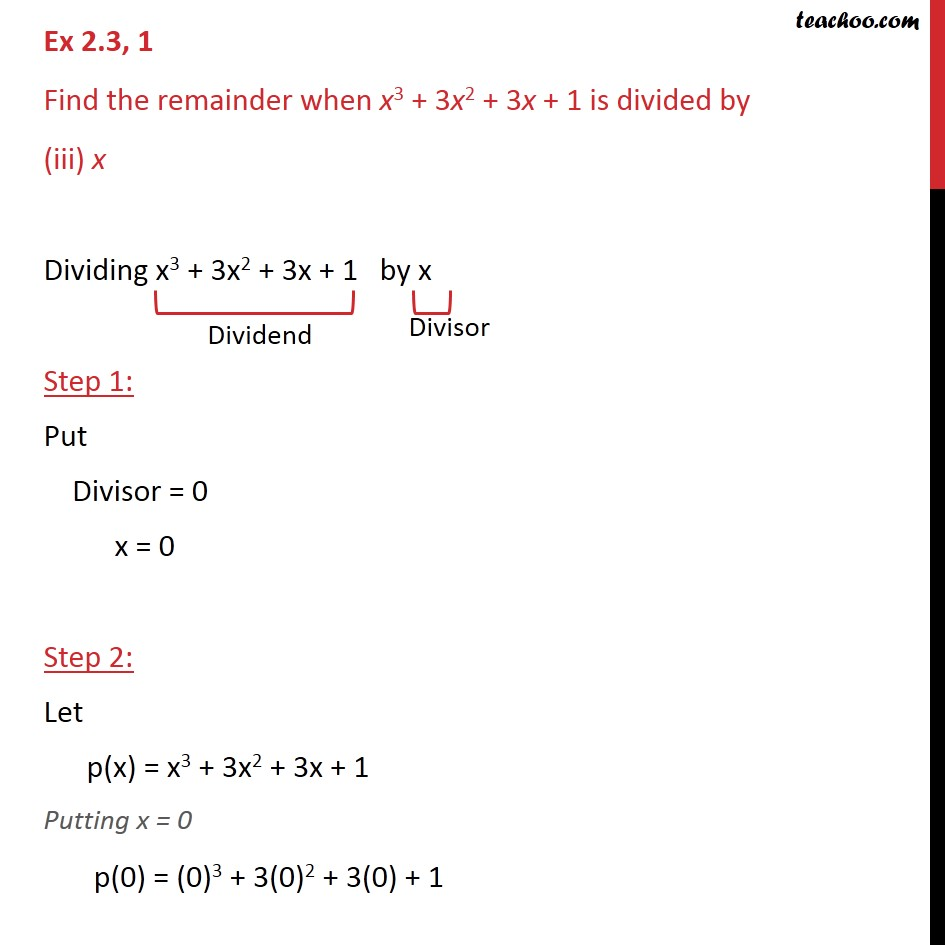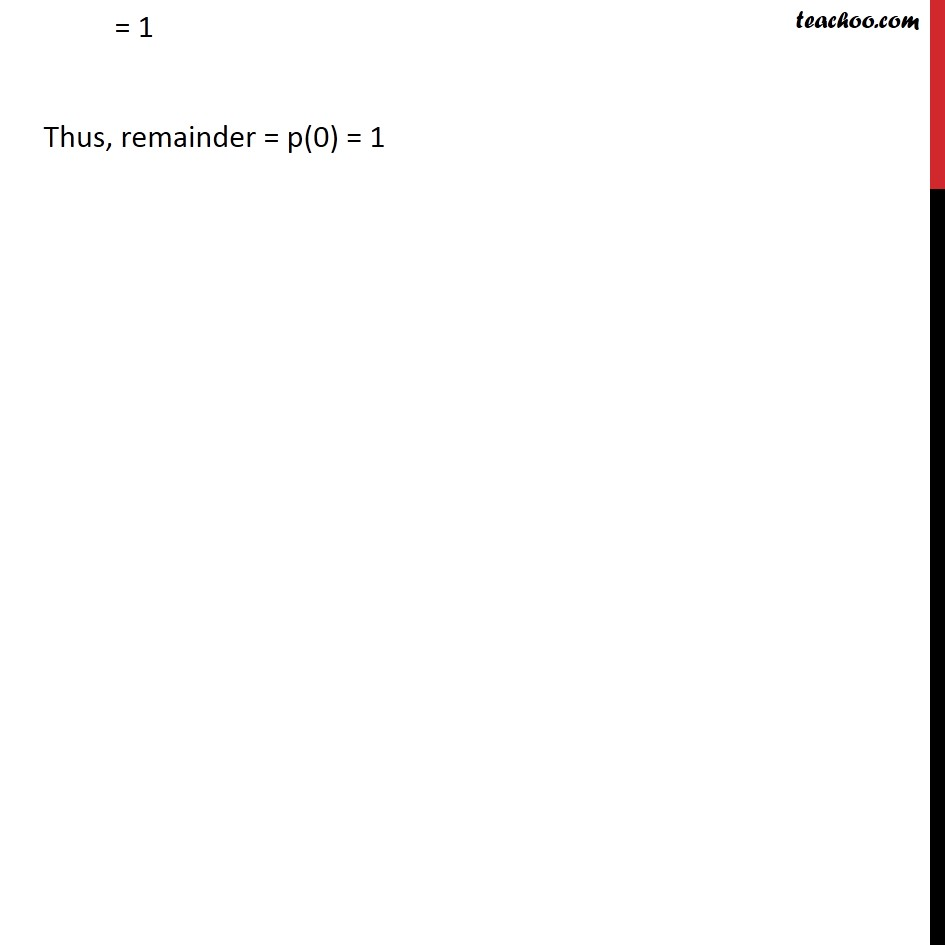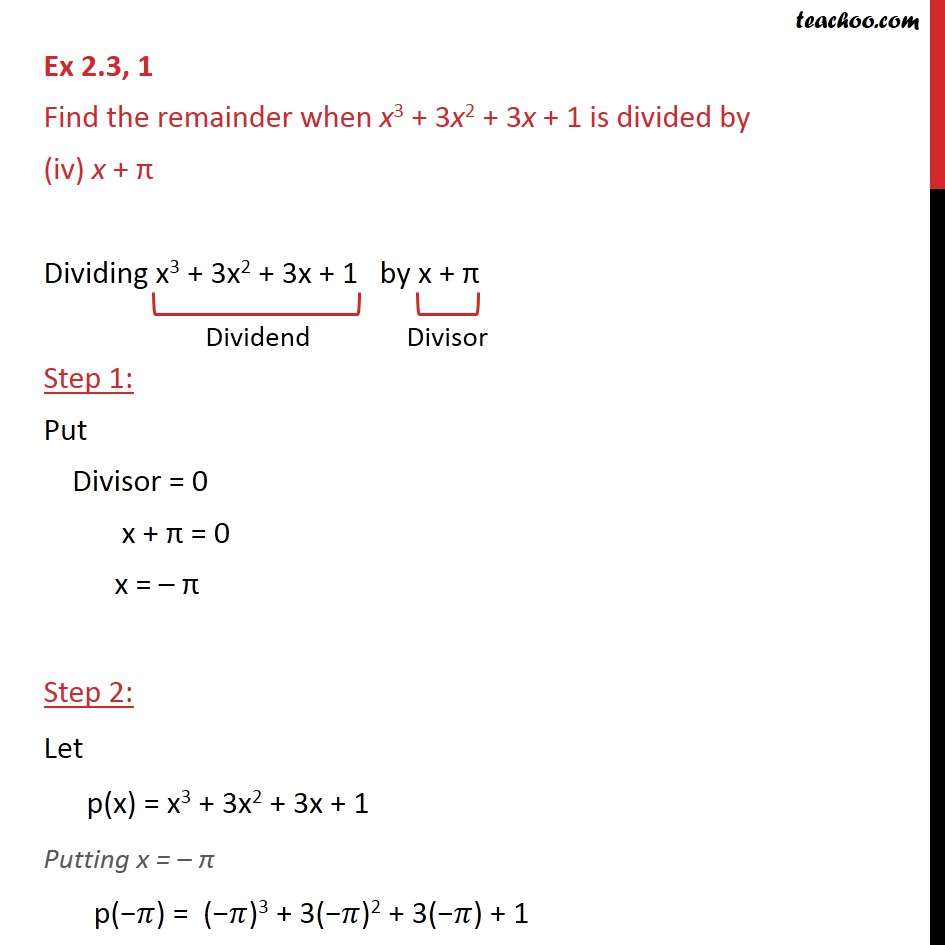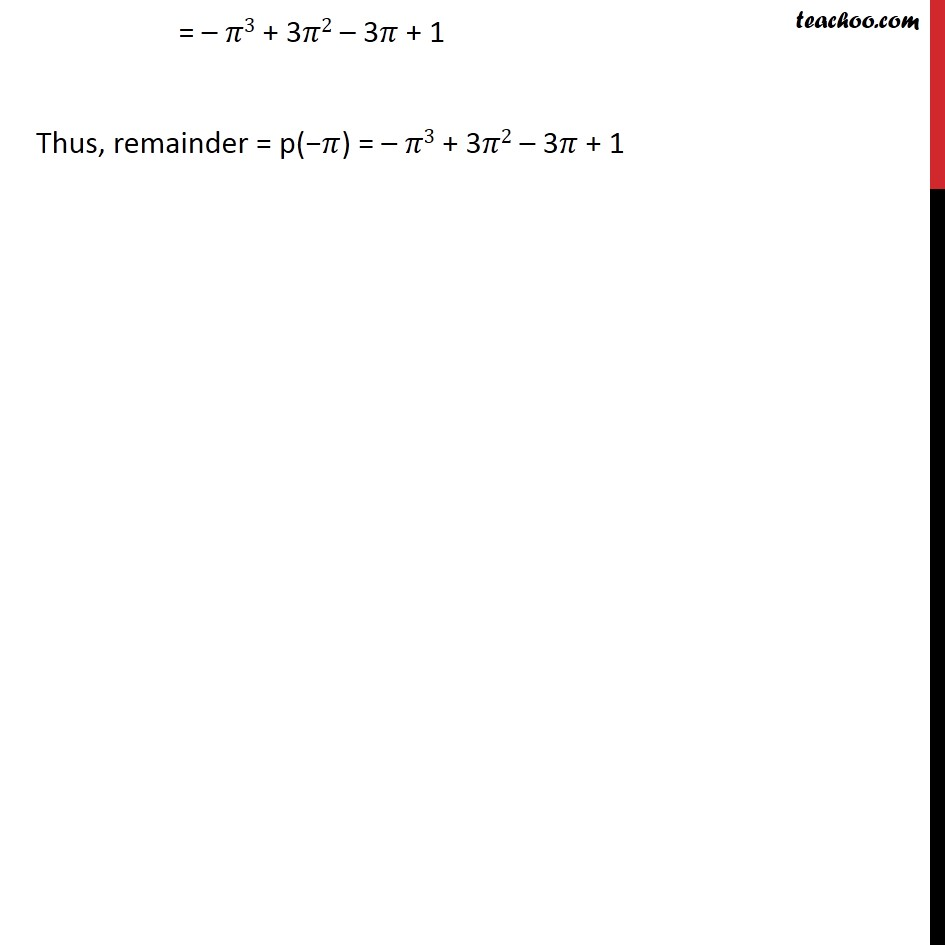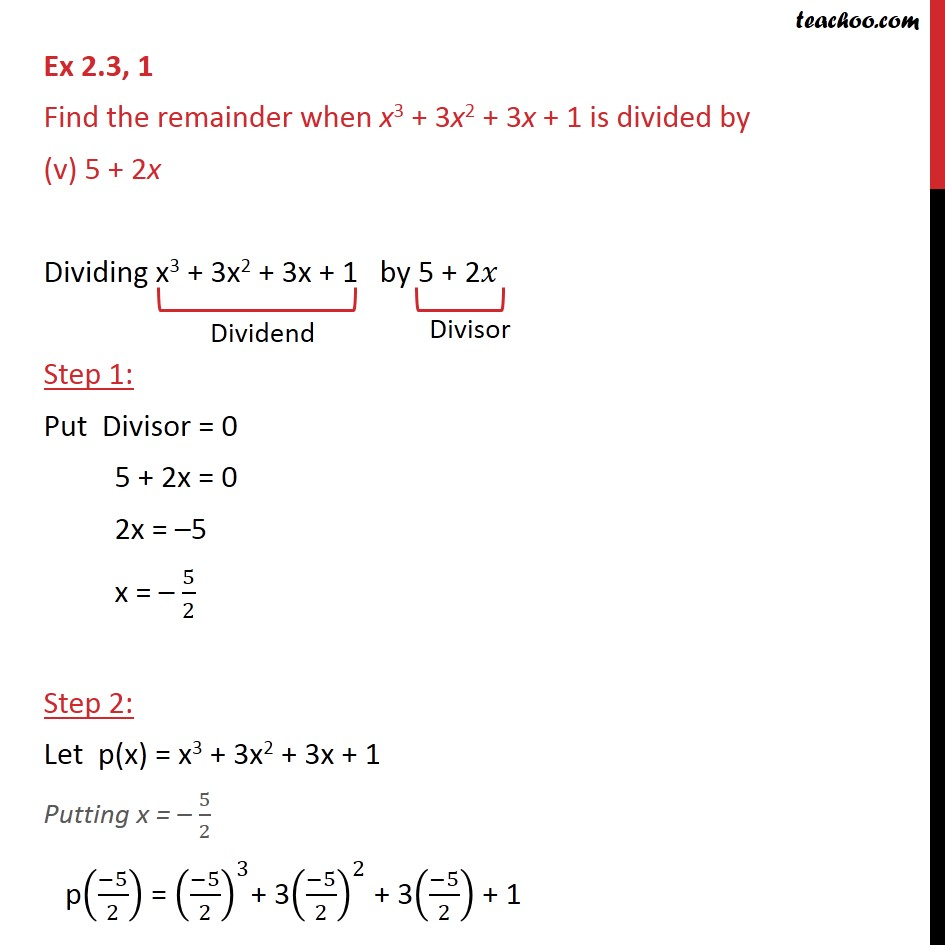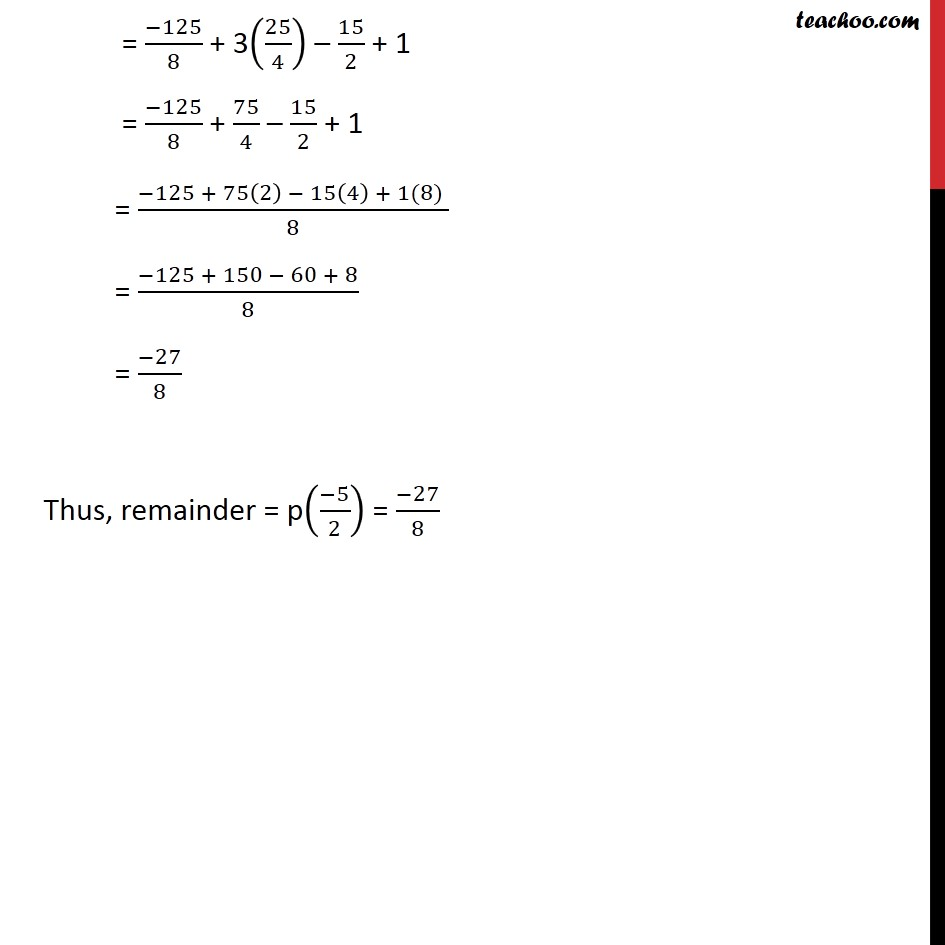1. Chapter 2 Class 9 Polynomials
2. Serial order wise

Transcript

Ex 2.3, 1 Find the remainder when x3 + 3x2 + 3x + 1 is divided by (i) x + 1 Dividing x3 + 3x2 + 3x + 1 by x + 1 Step 1: Put Divisor = 0 x + 1 = 0 x = –1 Step 2: Let p(x) = x3 + 3x2 + 3x + 1 Putting x = –1 p(–1) = (–1)3 + 3(–1)2 + 3(–1) + 1 = – 1 + 3 – 3 + 1 = 0 Thus, remainder = p(–1) = 0 Ex 2.3, 1 Find the remainder when x3 + 3x2 + 3x + 1 is divided by (ii) x – 1/2 Dividing x3 + 3x2 + 3x + 1 by 𝑥 – 1/2 Step 1: Put Divisor = 0 x – 1/2 = 0 x = 1/2 Step 2: Let p(x) = x3 + 3x2 + 3x + 1 Putting x = 1/2 p(1/2) = (1/2)^3+ 3(1/2)^2 + 3(1/2) + 1 = 1/8 + 3(1/4) + 3/2 + 1 = 1/8 + 3/4 + 3/2 + 1 = (1 + 3(2) + 3(4) +1(8))/8 = (1 + 6 + 1 2 + 8)/8 = 27/8 Thus, remainder = p(1/2) = 27/8 Ex 2.3, 1 Find the remainder when x3 + 3x2 + 3x + 1 is divided by (iii) x Dividing x3 + 3x2 + 3x + 1 by x Step 1: Put Divisor = 0 x = 0 Step 2: Let p(x) = x3 + 3x2 + 3x + 1 Putting x = 0 p(0) = (0)3 + 3(0)2 + 3(0) + 1 = 1 Thus, remainder = p(0) = 1 Ex 2.3, 1 Find the remainder when x3 + 3x2 + 3x + 1 is divided by (iv) x + π Dividing x3 + 3x2 + 3x + 1 by x + π Step 1: Put Divisor = 0 x + π = 0 x = – π Step 2: Let p(x) = x3 + 3x2 + 3x + 1 Putting x = – π p("−" 𝜋) = ("−" 𝜋)3 + 3("−" 𝜋)2 + 3("−" 𝜋) + 1 = – 𝜋3 + 3𝜋2 – 3𝜋 + 1 Thus, remainder = p("−" 𝜋) = – 𝜋3 + 3𝜋2 – 3𝜋 + 1 Ex 2.3, 1 Find the remainder when x3 + 3x2 + 3x + 1 is divided by (v) 5 + 2x Dividing x3 + 3x2 + 3x + 1 by 5 + 2𝑥 Step 1: Put Divisor = 0 5 + 2x = 0 2x = –5 x = – 5/2 Step 2: Let p(x) = x3 + 3x2 + 3x + 1 Putting x = – 5/2 p((−5)/2) = ((−5)/2)^3+ 3((−5)/2)^2 + 3((−5)/2) + 1 = (−125)/8 + 3(25/4) – 15/2 + 1 = (−125)/8 + 75/4 – 15/2 + 1 = (−125 + 75(2) − 15(4) + 1(8) )/8 = (−125 + 150 − 60 + 8)/8 = (−27)/8 Thus, remainder = p((−5)/2) = (−27)/8

Chapter 2 Class 9 Polynomials
Serial order wise